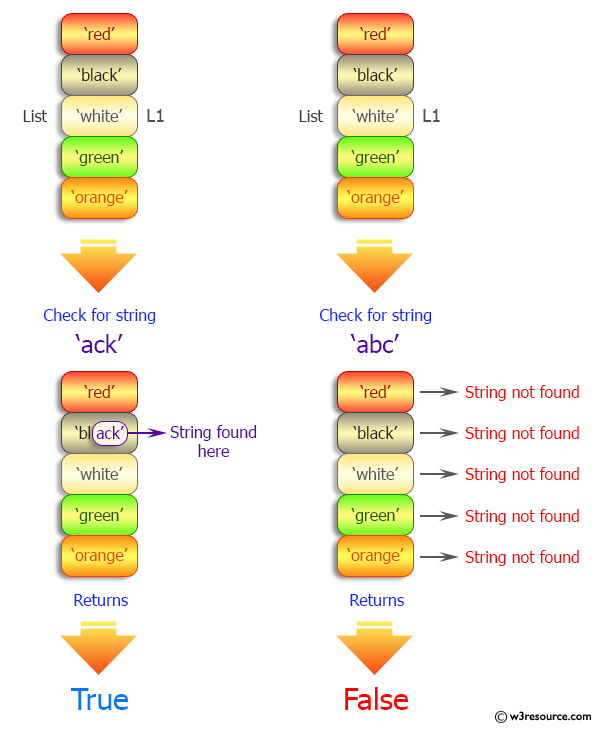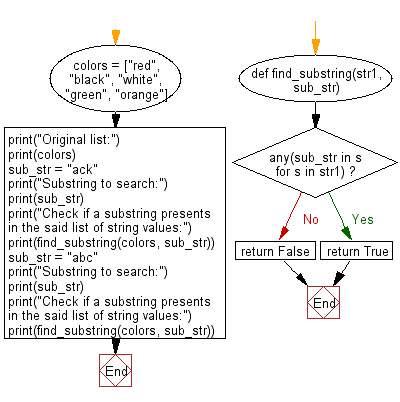﻿ Python: Check if a substring presents in a given list of string values - w3resource# Python: Check if a substring presents in a given list of string values

## Python List: Exercise - 119 with Solution

Write a Python program to check if a substring presents in a given list of string values.

Sample Solution:

Python Code:

``````def find_substring(str1, sub_str):
if any(sub_str in s for s in str1):
return True
return False

colors = ["red", "black", "white", "green", "orange"]
print("Original list:")
print(colors)
sub_str = "ack"
print("Substring to search:")
print(sub_str)
print("Check if a substring presents in the said list of string values:")
print(find_substring(colors, sub_str))
sub_str = "abc"
print("Substring to search:")
print(sub_str)
print("Check if a substring presents in the said list of string values:")
print(find_substring(colors, sub_str))
```
```

Sample Output:

```Original list:
['red', 'black', 'white', 'green', 'orange']
Substring to search:
ack
Check if a substring presents in the said list of string values:
True
Substring to search:
abc
Check if a substring presents in the said list of string values:
False
```

Pictorial Presentation:Flowchart:## Visualize Python code execution:

The following tool visualize what the computer is doing step-by-step as it executes the said program:

Python Code Editor:

Have another way to solve this solution? Contribute your code (and comments) through Disqus.

What is the difficulty level of this exercise?

Test your Python skills with w3resource's quiz

﻿

## Python: Tips of the Day

Floor Division:

When we speak of division we normally mean (/) float division operator, this will give a precise result in float format with decimals.

For a rounded integer result there is (//) floor division operator in Python. Floor division will only give integer results that are round numbers.

```print(1000 // 300)
print(1000 / 300)```

Output:

```3
3.3333333333333335```